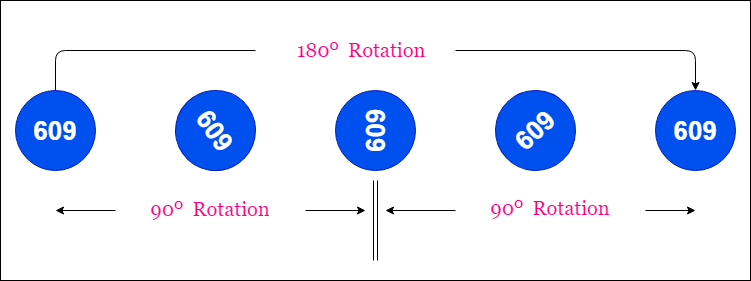## Check if a number is a Strobogrammatic number

A strobogrammatic number is a number that looks the same when rotated 180 degrees (looked at upside down). Write a function to determine if a number is strobogrammatic. The number is represented as a string.

According to Wikipedia "A strobogrammatic number is a number whose numeral is rotationally symmetric so that it appears the same when rotated 180 degrees.

Example 1:

```Input:  "69"
Output: true
```

Example 2:

```Input:  "88"
Output: true```

Example 3:

```Input:  "962"
Output: false```

The first few strobogrammatic numbers are:

```0, 1,  8, 11, 69, 88,  96, 101, 111, 181, 609,
619, 689, 808, 818, 888,  906, 916, 986, 1001,
1111,1691, 1881, 1961, 6009, 6119, 6699, 6889,
6969, 8008, 8118, 8698, 8888, 8968, 9006, 9116,
9696, 9886, 9966, ...```

### Check the following figure to get more clarification.Java Code:

```import java.util.HashMap;
import java.util.Map;

class StrobogrammaticNumber {

public static void main(String[] args) {
String number = "2323";
Solution solution = new Solution();
System.out.println(solution.isStrobogrammatic(number));
}
}

class Solution {

public boolean isStrobogrammatic(String num) {

if (num == null || num.length() == 0) {
return false;
}
Map<Character, Character> dictionary = new HashMap<>();
dictionary.put('0', '0');
dictionary.put('1', '1');
dictionary.put('6', '9');
dictionary.put('8', '8');
dictionary.put('9', '6');

char[] arr = num.toCharArray();
for (int i = 0, j = (arr.length - 1); i < j; i++, j--) {
char current = arr[i];
Character value = dictionary.get(current);
if (value == null) {
return false;
}
if (value != arr[j]) {
return false;
}
}
return true;
}
}```